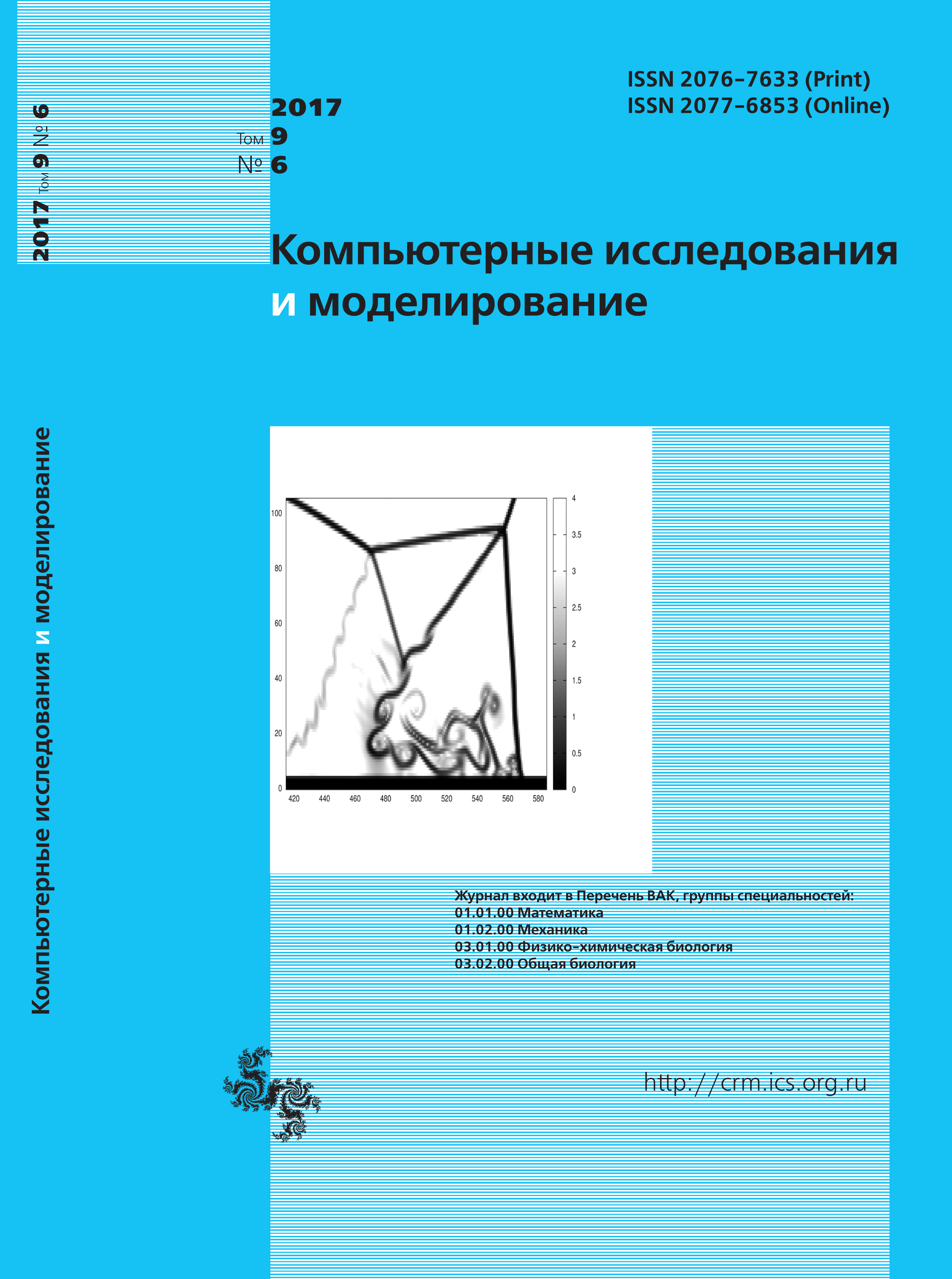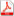Issue 6, 2017 Vol. 9

# All issues

Kinetic equations for modelling of diffusion processes by lattice Boltzmann methodpdf (1936K)  / List of references

The system of linear hyperbolic kinetic equations with the relaxation term of Bhatnagar–Gross–Krook type for modelling of linear diffusion processes by the lattice Boltzmann method is considered. The coefficients of the equations depend on the discrete velocities from the pattern in velocity space. The system may be considered as an alternative mathematical model of the linear diffusion process. The cases of widely-used patterns on speed variables are considered. The case of parametric coefficients takes into account. By application of the method of Chapman–Enskog asymptotic expansion it is obtained, that the system may be reduced to the linear diffusion equation. The expression of the diffusion coefficient is obtained. As a result of the analysis of this expression, the existence of numerical diffusion in solutions obtained by application of lattice Boltzmann equations is demonstrated. Stability analysis is based on the investigation of wave modes defined by the solutions of hyperbolic system. In the cases of some one-dimensional patterns stability analysis may be realized analytically. In other cases the algorithm of numerical stability investigation is proposed. As a result of the numerical investigation stability of the solutions is shown for a wide range of input parameters. The sufficiency of the positivity of the relaxation parameter for the stability of solutions is demonstrated. The dispersion of the solutions, which is not realized for a linear diffusion equation, is demonstrated analytically and numerically for a wide range of the parameters. But the dispersive wave modes can be damped as an asymptotically stable solutions and the behavior of the solution is similar to the solution of linear diffusion equation. Numerical schemes, obtained from the proposed systems by various discretization techniques may be considered as a tool for computer modelling of diffusion processes, or as a solver for stationary problems and in applications of the splitting lattice Boltzmann method. Obtained results may be used for the comparison of the theoretical properties of the difference schemes of the lattice Boltzmann method for modelling of linear diffusion.

Keywords: lattice Boltzmann method, diffusion, stability, dispersion
Citation in English: Krivovichev G.V. Kinetic equations for modelling of diffusion processes by lattice Boltzmann method // Computer Research and Modeling, 2017, vol. 9, no. 6, pp. 919-936
Citation in English: Krivovichev G.V. Kinetic equations for modelling of diffusion processes by lattice Boltzmann method // Computer Research and Modeling, 2017, vol. 9, no. 6, pp. 919-936
DOI: 10.20537/2076-7633-2017-9-6-919-936
Views (last year): 25.

Full-text version of the journal is also available on the web site of the scientific electronic library eLIBRARY.RU

The journal is included in the Russian Science Citation Index

The journal is included in the List of Russian peer-reviewed journals publishing the main research results of PhD and doctoral dissertations.

International Interdisciplinary Conference "Mathematics. Computing. Education"

The journal is included in the RSCI

Indexed in Scopus